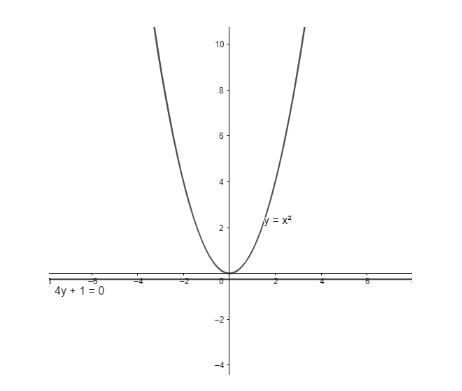Courses
Courses for Kids
Free study material
Free LIVE classes
MoreLIVE
Join Vedantu’s FREE Mastercalss

# The equation to the locus of the points from which the parabola, $y={{x}^{2}}$ can be seen at right angles is(a) $4x+1=0$(b) $4y-1=0$(c) $4y+1=0$(d) $y+4=0$Verified
362.4k+ views
Hint: The equation of locus of points from which the parabola can be seen at right angles is the equation of the director circle of the parabola. For a parabola, the equation of the directrix is the equation of the director circle of the parabola.

We have a parabola of the form $y={{x}^{2}}$.
We want to find the locus of points from which the parabola can be seen at right angles, i.e., the locus of points from which perpendicular tangents can be drawn to the parabola.
We know that the locus of points of intersection of the perpendicular tangents to a curve is called the director circle of the curve.
For a parabola, the equation of the director circle is the equation of the directrix of parabola.
We know that the directrix is a straight line, but it can be considered as a circle with infinite radius.The equation of directrix of parabola of the form ${{x}^{2}}=4ay$ is $y+a=0$.
Comparing it with the equation of our parabola, we get $a=\dfrac{1}{4}$
Substituting the above value in the equation $y+a=0$, we get $y+\dfrac{1}{4}=0$.
Rearranging the terms, we get
$\Rightarrow 4y+1=0$, which is the desired equation.
However, it is not necessary that we will always get the locus of intersection of tangents as a straight line. If the angle between the two tangents changes, the equation of locus of their point of intersection will also change depending upon the angle between the two tangents.
Hence, the correct answer is (c) $4y+1=0$.
Note: There’s no need to solve the question by finding the exact equations of tangents which are perpendicular to each other and then solve them to get the locus of their point of intersection. It will lead to a time consuming and longer solution.
Last updated date: 27th Sep 2023
Total views: 362.4k
Views today: 3.62k